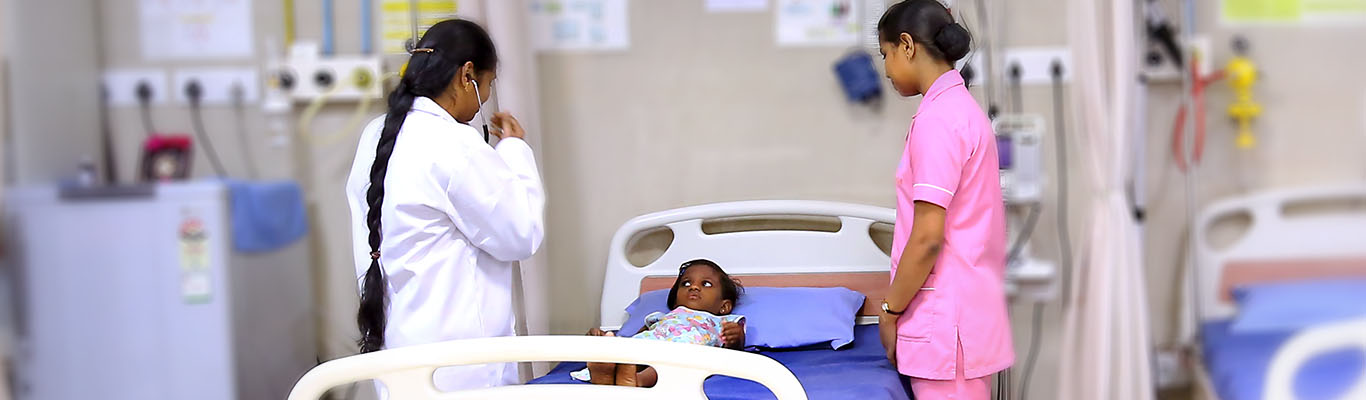Department Of
Paediatrics
+91 73583 56 333 +91 73583 17 333
SELECT * FROM doctors where IsDeleted=0 And (did<10000) And (speciality like '%Paediatrics%' OR subspeciality like '%Paediatrics%') ORDER BY CASE WHEN `did` = 2928 THEN 1 WHEN `did` = 1089 THEN 2 WHEN `did` = 1034 THEN 3 WHEN `did` = 2215 THEN 4 WHEN `did` = 1919 THEN 5 WHEN `did` = 1698 THEN 6 WHEN `did` = 1636 THEN 7 WHEN `did` = 1288 THEN 8 WHEN `did` = 1732 THEN 9 WHEN `did` = 1130 THEN 10 WHEN `did` = 2552 THEN 11 WHEN `did` = 1894 THEN 12 WHEN `did` = 2410 THEN 13 WHEN `did` = 1755 THEN 14 WHEN `did` = 2792 THEN 15 WHEN `did` = 2913 THEN 16 WHEN `did` = 2224 THEN 17 WHEN `did` = 1948 THEN 18 WHEN `did` = 1657 THEN 19 WHEN `did` = 2046 THEN 21 WHEN `did` = 3087 THEN 22 WHEN `did` = 3088 THEN 23 WHEN `did` = 3101 THEN 24 WHEN `did` = 3126 THEN 25 END;